Just the Math Please!
How many cubes?
Hahaha! Math!
Gimme that Cylinder!
Level Up!
100

A box has that is 10 inches long, 5 inches wide and 2 inches high.  What is the volume?

100 in3

100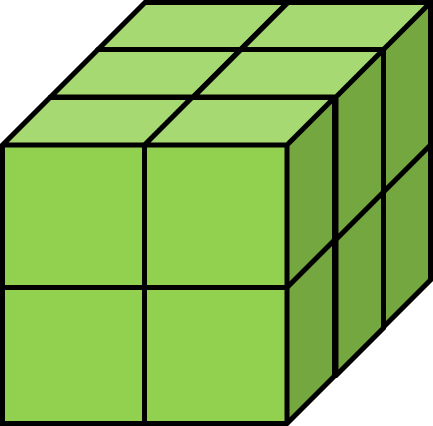2 x 3 x 2=12 units3

100

Why is the math book so sad?

Because it had so many problems!100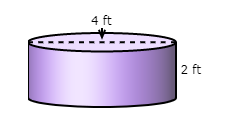What is the volume of this cylinder?

Use 𝜋 ≈ 3.14 and round your answer to the nearest hundredth.

25.12 ft³

100

A box has a volume of 48in3, a length of 3in and a width of 4in. What is the height?

height = 4in.

200

A box has that is 2 inches long, 8 inches wide and 1 inches high.  What is the volume?

16in3

2005 x 2 x 2=20 units3

200

Why can't a nose be 12 inches long?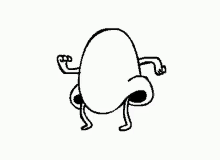Because then it would be a foot!200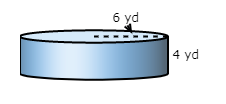What is the volume of this cylinder?

Use 𝜋 ≈ 3.14 and round your answer to the nearest hundredth.

452.16 yd³

200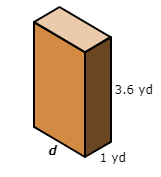The volume of this rectangular prism is 7.2 cubic yards. What is the value of d?

d = 2 yards
300

A box has that is 3 feet long, 3 feet wide and 3 feet high.  What is the volume?

27ft3

300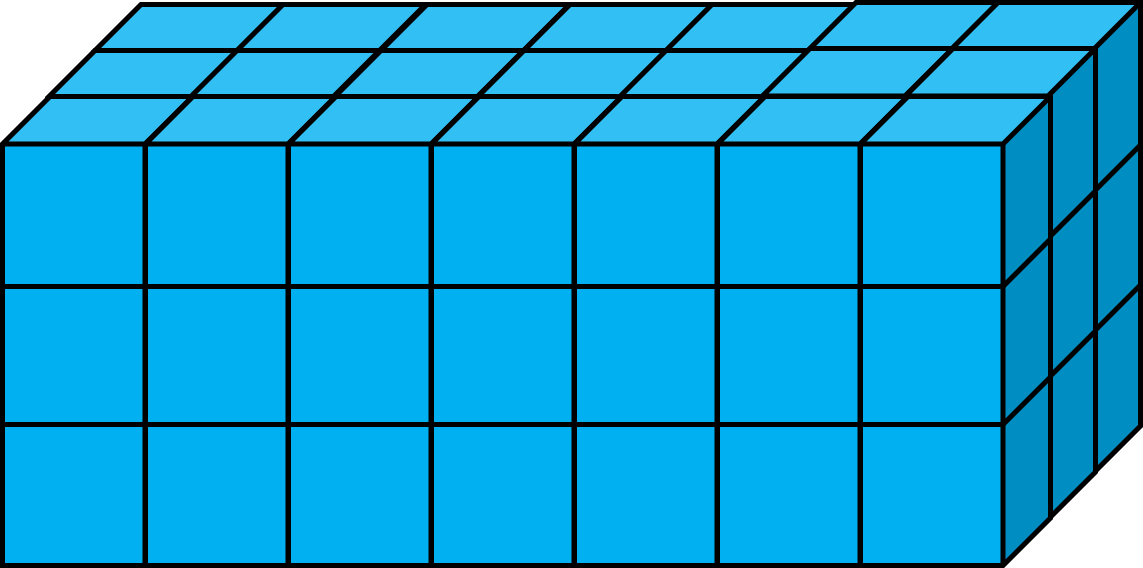7 x 3 x 3=63 units3

300

Why was 6 afraid of 7?Because 7, 8, 9!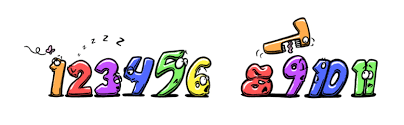300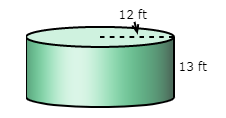What is the volume of this cylinder?

Use 𝜋 ≈ 3.14 and round your answer to the nearest hundredth.

5,878 ft3

300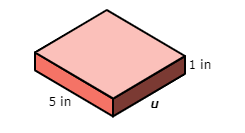The volume of this rectangular prism is 23.0 cubic inches. What is the value of u?

u = 4.6 inches

400

A box has that is 4 cm long, 6 cm wide and 5 cm high.  What is the volume?

120cm3

400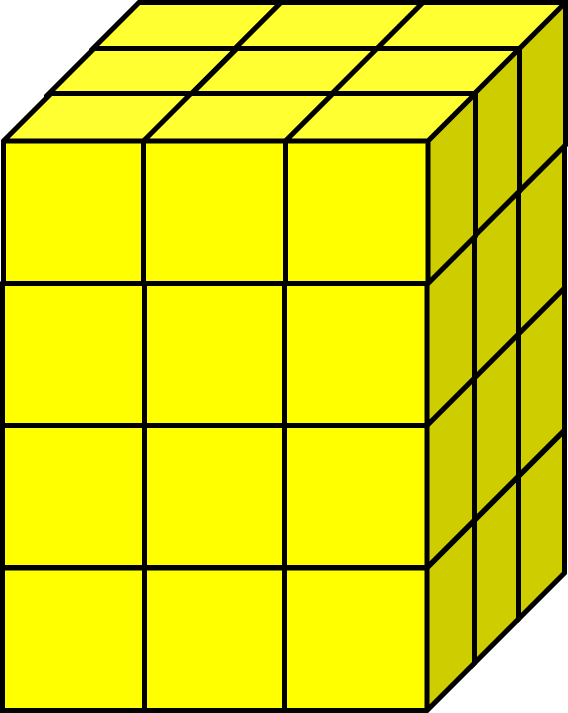3 x 3 x 4=36 units3

400

Are monsters good at math?

Not unless you Count Dracula!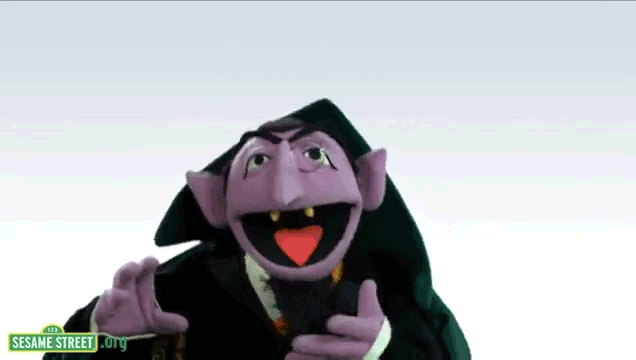400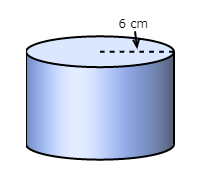The volume of the cylinder is 1,130.4. What is the height of this cylinder?

Use 𝜋 ≈ 3.14 and round your answer to the nearest hundredth.

10 cm³

400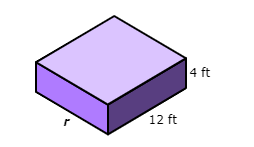The volume of this rectangular prism is 528 cubic feet. What is the value of r?

r = 11 feet

500

A box has that is 8 inches long, 5 inches wide and 10 inches high.  What is the volume?

400in3

500length = 8

height = 6

width = 16

576 units3

500

How do you make the number seven even?Remove the "s"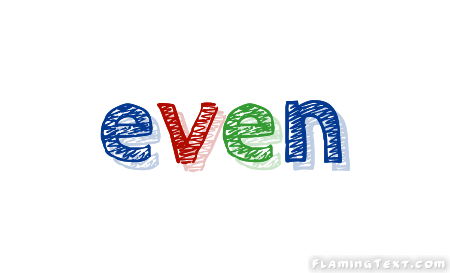500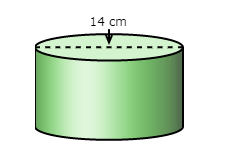The volume of the cylinder is 1,538.6. What is the height of this cylinder?
Use 𝜋 ≈ 3.14 and round your answer to the nearest hundredth.

10 cm³

500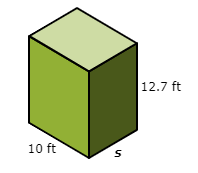The volume of this rectangular prism is 1,016.0 cubic feet. What is the value of s?

s = 8 feet

Click to zoom